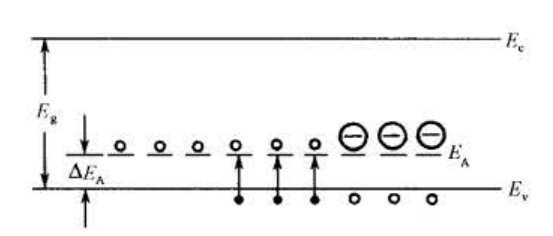«

## 使用声明

• 此内容由本人整理并参考了其他人整理的结果以及学术部大礼包，主要内容来自书本、个人理解和我手头上资料中阐述比较好的，由于个人水平有限，使用时请轻喷。
• 第一部分我尝试把相关的性质和内容一并捧上，但是实际上作为概念阐释的题，如果时间不够仅需记忆粗体的部分
• 祝各位能在期末拥有不错的成绩

## 一 概念解释(书上出现顺)

1. 禁带宽度$E_g$（P16）

脱离共价键所需的最低能量就是禁带宽度

2. 本征激发（P16，P75）

当处于$T>0$K的一定温度下，共价键上的电子依靠热激发有可能获得能量而脱离共价键，在晶体中自由运动，成为准自由电子，即价带电子激发成为导带电子，同时价带中产生了空穴，这个过程称为本征激发

3. 有效质量$m_n^*$（P19）

$$m_n^*=\frac{\hbar^2}{\frac{d^2 E}{dk^2}}$$

1. 在有外电场的情况下，半导体中的电子所受外力利用有效质量可以得到和牛二律相同的形式（$a=\frac{f}{m_n^*}$）
2. 有效质量可以直接由实验测定
3. 引入有效质量可以使得计算变简单而不需要考虑除外力外电子收到原子和其他电子势场的影响
4. 是原子核周期性势场和其他电子势场的综合影响结果
4. 空穴（P21）
1. 空穴是价带中空着的状态，通常被看成是带正电的粒子
2. 空穴有正电荷$+q$，也有正的有效质量($m_p^*$)
5. 间隙杂质（P46）

间隙杂质是指杂质原子位于晶格原子间空隙的位置

6. 受主能级$E_A$（P48）

III族杂质在硅或锗中能够接受电子而产生导电空穴，形成一个负电中心，把这样的受主杂质束缚在空穴的能量状态称为受主能级。空穴需要$\Delta E_A$的能量才能挣脱受主杂质的束缚成为导电空穴。

7. 杂质补偿作用（P50）

当一个半导体中同时存在施主和受主杂质，两者之间存在相互抵消的作用，称为杂质的补偿作用。

8. 深能级杂质（P51）
1. 非III、V族的杂质在硅或锗的禁带中产生的施主能级距离导带底较远，他们产生的施主能级距离导带顶也较远，这种能级被称为深能级，且相应的杂质称为深能级杂质
2. 深能级杂质可以产生多次电离，每次电离都会对应一个能级，可以引入若干个施主能级或受主能级。
9. 费米能级$E_F$（P69）
1. 费米能级是系统的化学势$E_F=\mu=\left(\frac{\partial F}{\partial N}\right)_T$，式中的$F$为系统的自由能
2. 当系统处于热平衡状态，也不对外界做功的情况选，系统中增加一个电子所引起的系统自由能的变化等于系统的费米能级。
3. 处于热平衡状态的系统有统一的费米能级，在一定的温度下只要知道了费米能级就可以确定电子在各量子态上的统计分布。
4. 费米分布函数$f(E)=\frac{1}{1+e^\frac{E-E_F}{k_0T}}$
10. 本征半导体（P75）

本征半导体就是一块没有杂质和缺陷的半导体

11. 简并半导体（P93）

当n型半导体的杂质掺杂水平很高（$N_D$很大），费米能级会进入导带，导带底被电子占据，当p型半导体的杂质掺杂水平很高（$N_A$很大），费米能级会进入价带，价带顶被空穴占据，此时不能在应用波兹曼分布函数而需要考虑泡利不相容原理，利用费米分布函数来分析导带中电子及价带中空穴的统计分布问题，这种情况称为载流子的简并化，发生这种简并化的半导体为简并半导体。

12. 载流子迁移率（P107）

当导体内部电场恒定的时候，载流子有一个恒定的平均漂移速度，其与电场成正比，$\bar{v}_d=\mu E$，式中的$\mu$为载流子的迁移率，表示单位场强下载流子的平均漂移速度

13. 平均自由程（P109）

自由载流子连续两次散射之间的自由运动平均路程为平均自由程

14. 热载流子（P132）

在强电场下，载流子从电场中获得很多能量，使得其平均能量大于热平衡时的能量，从而载流子和晶格系统不处于热平衡状态，这种状态下的载流子称为热载流子

15. 非平衡载流子（P145）

当对于半导体施加外界作用，破坏了热平衡条件时，其处于一个与热平衡相偏离的非平衡状态，在这种状态下的载流子浓度不再是$n_0$和$p_0$，而会多出来一部分，这比平衡状态多出来的部分即是非平衡载流子

16. 少子寿命（P147）

当光照停止时，会产生非平衡载流子的复合，$\Delta p$随时间指数减少，存在一个消失的过程，在导带和价带中有一定的生存时间，非平衡载流子的平均生存时间称为非平衡载流子的寿命。因为相对于非平衡多数载流子，非平衡少数载流子对于其平均生存时间起决定性影响，因此非平衡载流子的寿命常称为少数载流子寿命

17. 准费米能级（P148）

当半导体中的电子系统处于热平衡状态时，整个半导体系统拥有统一的费米能级，但是当外界因素破坏了热平衡，此时就不存在统一的费米能级。由于电子系统的热平衡时通过热跃迁实现的，在同一个能带中热跃迁十分频繁，但是在两个能带中隔着禁带热跃迁十分稀少，可以认为价带和导带中国的电子各自基本处于平衡态，而价带和导带之间处于非平衡状态，因此对导带和价带费米能级和统计规律仍是适用的，可以引入局部的费米能级，这种费米能级称为准费米能级

18. 复合中心（P150、152）

促进复合过程的杂质和缺陷就是复合中心。半导体中的杂质越多、晶格缺陷越多，寿命越短，其中杂质和缺陷有促进复合的作用

19. 间接复合（P150、152）

非平衡载流子通过复合中心复合

20. 俄歇复合（P150、159）

载流子从高能级向低能级跃迁，发生电子——空穴复合时，把多余的能量传给另一个载流子，使这个载流子被激发到能量更高的能级上，当它重新跃迁回低能级时，多余的能量通常以声子放出，这种复合形式称为俄歇复合

21. 陷阱效应（P162）
1. 是在有非平衡载流子的情况下发生的一种效应
2. 当平衡破坏的时候，能级有收容非平衡的电子和空穴的能力，这种积累非平衡载流子的作用叫做陷阱效应
3. 当杂质能级与平衡时费米能级重合时，最有利于陷阱作用
22. 扩散长度$L_p,L_n$（P166）
1. 扩散长度标志着非平衡载流子深入样品的平均距离
2. 扩散长度由扩散系数和材料的寿命决定
23. pn结（P181）

在p型或n型半导体中通过一定工艺把n型或p型杂质掺入其中，使得不同的区域有n型和p型的导电类型，在两种导电类型的交界面处产生了pn结

24. 本征吸收（P317）

理想半导体在绝对零度时价带被电子占满，不会激发到更高的能级。当吸收足够能量的光子可以使得电子激发，越过禁带跃迁入空的导带，从而留下空穴形成电子-空穴对。这种由于电子在带与带之间的跃迁所形成的吸收过程时本征吸收

## 二 主要物理问题

1. 试说明：本征半导体材料中的载流子浓度随禁带宽度$E_g$及温度$T$的变化情况，并解释原因。

本征半导体的载流子浓度为$n_i=n_0=p_0=\sqrt{N_cN_v}e^{-\frac{E_g}{2k_0T}}$
禁带宽度变宽、温度下降则本征半导体材料中的载流子浓度下降，反之上升。
温度： 随温度升高，晶格震动剧烈，内部电子与晶格的碰撞概率增加，吸收声子的可能性增加，能量更强，从而内部电子从价带跃迁到导带的几率增加，载流子浓度增加。
禁带宽度： 禁带宽度越宽，载流子跃迁需要克服更多的能量，跃迁概率降低，载流子浓度减小。

2. 写出爱因斯坦关系式，分析扩散系数受哪些因素影响。

爱因斯坦关系式：$\left\{\begin{array}{l}\text{电子:}\frac{D_{\mathrm{n}}}{\mu_{\mathrm{n}}}=\frac{k_{0} T}{q} \\ \text{空穴:}\frac{D_{\mathrm{p}}}{\mu_{\mathrm{p}}}=\frac{k_{0} T}{q}\end{array}\right.$
扩散系数$D$受到温度和载流子迁移率$\mu$控制，当温度越高，扩散系数越大，当载流子迁移率越小，扩散系数越小
其中迁移率受到半导体材料、杂质浓度和杂质类型影响，则这些因素也间接影响扩散系数。

3. 半导体中寿命指什么？它与何机制有关？试说明影响寿命的相关因素。

寿命指的是非平衡载流子的平均生存时间
主要与各种复合机制有关，如直接复合和间接复合等
主要的影响因素有：

1. 复合中心的情况，如载流子浓度、能级、俘获截面
2. 半导体本身的性质，如半导体杂质类型、杂质浓度、晶格缺陷
3. 平衡载流子的浓度影响，如禁带宽度、温度
4. 跃迁几率的影响，如载流子的速度、浓度、温度等
5. 非平衡载流子的浓度
4. 常见半导体中的载流子两主要来源是什么？它们随温度的变化情况如何？在何情况下各占主要地位，并以此说明半导体器件存在极限工作温度的原因。

常见的导体中载流子主要来源于杂质电离和本征激发 ，在本征半导体中只有本征激发，而在一般半导体中主要来源于杂质电离，在低温时可以忽略本征激发，而当温度增高，杂质半导体中的本征载流子迅速增加而使得器件无法正常工作。
对于一般半导体中，当温度较低时，本征载流子浓度较低，此时杂质能级更接近允带，因此更容易跃迁到允带中，故在杂质发生强电离之前，杂质电离占主要地位。之后，杂质发生强电离，并逐渐进入过渡区后，温度进一步升高，本征激发将占主导地位。
由于本征激发载流子浓度随温度变化剧烈，而半导体器件在工作过程中都会发热，因而由本征主导的器件工作极不稳定，故温度增加到一定程度后，本征激发将占主导地位，此时器件无法稳定工作，此即器件的存在极限工作温度的原因。
总结：低温以杂质电离为主，高温以本征激发为主

5. 简单说明p-n结的作用和用途。

1. p-n结中由于空间电荷区的作用，形成对电子运动有阻碍的势垒。载流子通过这个势垒时，按他的运动方向有难有易，利用这种现象的就是二极管
2. 当光照射半导体时，产生电子空穴对；因此电导率增加，但不形成电流。但是在p-n结中有自建电场，载流子分布也不均匀，所以在pn结中光照射形成电流（光电流），这里的光包括放射线，因此利用这种现象的器件有光电池，放射线，红外线探测
3. p-n结两边存有正负电荷，而且随偏压的大小变化，也就是说pn结具有静电容，这些电容与偏压有关，利用这种关系的成为变容二极管
4. p-n结厚度随各区杂质密度的不同而不同，如果杂质密度高，结厚度就薄，容易引起电子的隧道效应，这时伏安特性曲线与一般二极管不同，有所谓负阻特性，这种二极管成为隧道二极管，常用于振荡器、放大器等
5. 当p-n结上有压力时，电导率有明显的变化，利用这种原理可以制成压力传感器
6. 漂移运动和扩散运动有什么不同？漂移运动与扩散运动之间有什么联系？非简并半导体的迁移率与扩散系数之间有什么联系 。

漂移运动是载流子在外电场的作用下发生的定向运动，而扩散运动是由于浓度分布不均匀导致载流子从浓度高的地方向浓度低的地方向的定向运动，前者的推动力是外电场，后者的推动力则是载流子分布的浓度梯度。少数载流子漂移正是因为多数载流子扩散运动产生的内电场而引起的。
非简并半导体迁移率与扩散系数：爱因斯坦关系

7. 试定性分析Si的电阻率与温度的变化关系。

电导率的表达公式为$\sigma=q(n\mu+p\mu)$
电阻率变化主要有以下三个过程

1. 温度很低时，电阻率随温度升高而降低，因为这时本征激发极弱，可以忽略。载流子主要来源于杂质电离，随着温度升高，载流子浓度逐步增加，相应地电离杂质散射也随之增加，从而使得迁移率随温度升高而增大，导致电阻率随温度升高而降低，
2. 温度进步增加(含室温)，电阻率随温度升高而升高。在这一温度范围内，杂质已经全部电离，同时本征激发尚不明显，故载流子浓度基本没有变化。对散射起主要作用的是晶格散射，迁移率随温度升高而降低，导致电阻率随温度升高而升高
3. 温度再进一步增加，电阻率随温度升高而降低。这时本征激发越来越多，虽然迁移率随温度升高而降低，但是本征载流子增加很快，其影响大大超过了迁移率降低对电阻率的影响，导致电阻率随温度升高面降低。当然，温度超过器件的最高工作温度时，器件已经不能正常工作了
8. 间接复合是半导体中一种很重要的复合机制，它描述了载流子通过杂质能级在各允带间跃迁情况，试描述该机制的四个基本跃迁过程，并写出每过程中载流子的变化率表达式（产生率或俘获率，需要的参数自己假设）

 过程 变化率 1 俘获电子过程 电子俘获率 $r_nn(N_t-n_t)$ 2 发射电子过程 电子产生率 $r_nn_1n_t,n_1=N_ce^{\frac{E_t-E_c}{k_0T}}$ 3 俘获空穴过程 空穴俘获率 $r_ppn_t$ 4 发射空穴过程 空穴产生率 $r_pp_1(N_t-n_t),p_1=N_ve^{-\frac{E_t-E_v}{k_0T}}$

9. 写出电子和空穴的连续性方程，并任选一方程说明其中各项所代表的意义及该方程成立的原因（注意对各项正负号的说明）。

电子 $\frac{\partial n}{\partial t}=\frac{1}{q} \nabla \cdot \boldsymbol{J}_{\mathrm{n}}-\frac{\Delta n}{\tau_{\mathrm{n}}}+g_{\mathrm{n}}$
空穴 $\frac{\partial p}{\partial t}=-\frac{1}{q} \nabla \cdot \boldsymbol{J}_{\mathrm{p}}-\frac{\Delta p}{\tau_{\mathrm{p}}}+g_{\mathrm{p}}$
第一项为电流所引起的载流子在单位体积中的积累率，这一点由负的电流密度的散度决定，因此电子取正，空穴取负
第二项为当光照均匀时非平衡载流子衰减的微分表达式，因为是衰减，取负
最后一项为产生率，因此取正

10. 对于N型半导体，在杂质饱和电离和本征激发共存温度范围：
1. 写出电中性条件
2. 导出电子浓度表达方式
1. $p+n_D^+=n$
2. 由于杂质饱和电离，$n_D^+\approx N_D$
利用$np=n_i^2$，上式可改写为$n^2-N_Dn-n^2_i=0$
从而可以得到电子的浓度为
$n=\frac{N_D}{2}(1+\sqrt{1+\frac{4n_i^2}{N_D^2}})$
11. 什么叫受主？什么叫受主电离？受主电离前后有何特征？试举例说明之，并用能带图表征出p型半导体
1. 受主是指III族杂质在硅或锗中可以接受电子而形成导电空穴，并形成负电中心，即受主杂质
2. 受主电离是指使空穴挣脱受主杂质束缚成为导电空穴的过程
3. 受主电离后使得价带中导电空穴增多，增强半导体的导电性
例子： 硅晶体中掺硼，一个硼原子占据了硅原子的位置。硼原子有3个价电子，当它和 周围的四个硅原子形成共价键时，还缺少一个电子，必须从别处的硅原子中夺取一个价电 子，于是从硅晶体的共价键中产生了一个空穴。而硼原子接受一个电子后，成为带负电的 硼离子，称为负电中心。12. 试分别定性定量说明：
1. 在一定的温度下，对本征材料而言，材料的禁带宽度越窄，载流子浓度越高；
2. 对一定的材料，当掺杂浓度一定时，温度越高，载流子浓度越高。
1. 本征半导体的载流子浓度为$n_i=n_0=p_0=\sqrt{N_cN_v}e^{-\frac{E_g}{2k_0T}}$
可以看到当温度相同时，材料的禁带宽度越窄载流子浓度越高。
2. 当掺杂浓度一定时，当温度升高，杂质会从弱电离到中间电离区到最后强电离区，费米能级先升后大幅下降，施主杂质电离更加彻底载流子浓度不断提高直至施主杂质完全电离。
$$\left\{\begin{array}{l}n_0=N_ce^{-\frac{E_c-E_F}{k_0T}}=\frac{2(2\pi m_n^*k_0T)^\frac{3}{2}}{h^3}e^{-\frac{E_c-E_F}{k_0T}}\\p_0=N_ve^{-\frac{E_F-E_v}{k_0T}}=\frac{2(2\pi m_p^*k_0T)^\frac{3}{2}}{h^3}e^{-\frac{E_F-E_v}{k_0T}}\end{array}\right.$$
当温度进一步升高，本征激发的载流子同1中逐渐占领主导且随温度增加。
13. 掺杂半导体与本征半导体之间有何差异？试举例说明掺杂对半导体的导电性能的影响。

在纯净的半导体中掺入杂质后，可以控制半导体的导电特性。掺杂半导体又分为n型半导体和p型半导体。
例如，在常温情况下，本征Si中的电子浓度和空穴浓度均为1.5$\times$10^10^cm^−3^，当在Si中掺入1.0$\times$10^16^cm^−3^（As等）后，半导体中的电子浓度将变为1.0$\times$10^16^cm^−3^,而空穴浓度将近似为2.25$\times$10^4^cm^−3^。半导体中的多数载流子是电子，而少数载流子是空穴

14. 证明非平衡载流子的寿命满足$\Delta p(t)=\Delta p_{0} e^{-\frac{t}{\tau}}$，并说明式中各项的物理意义。

单位时间内非平衡载流子的减少数为$-\frac{d \Delta p(x)}{d t}$
而单位时间内复合的非平衡载流子数为$\frac{\Delta p}{\tau}$
如果在$t=0$时刻撤除光照，则在单位时间内非平衡载流子的减少数=单位时间内复合的非平衡载流子数
$\frac{-d \Delta p(x)}{d t}=\frac{\Delta p}{\tau}$
对两边积分即可得到$\Delta p(t)=\Delta p_{0} e^{-\frac{t}{\tau}}$
其中：
$\tau$为载流子寿命，即非平衡载流子的平均生存时间
$\frac{\Delta p}{\tau}$为复合几率，即复合作用下载流子因复合而导致单位时间，单位体积内载流子的减少数
$\Delta p_0$积分常数，其物理意义为t=0时刻即初始状态下的非平衡载流子数目

15. 以As掺入Ge中为例，说明什么是施主杂质、施主杂质电离过程和n型半导体。

As有5个价电子，其中的四个价电子与周围的四个Ge院子形成共价键，还剩余一个电子，同时As原子所在处也多于一个正电荷，称为正离子中心，所以，一个As原子取代一个Ge原子，其效果是形成一个正电中心和一个多余的电子，多余的电子束缚在正电中心，但这种束缚很弱，很小的能量就可使电子摆脱束缚，称为在晶格中导电的自由电子，而As原子形成一个不能移动的正电中心。这个过程叫做施主杂质的电离过程。能够释放电子而在导带中产生电子并形成正电中心，称为施主杂质或N型掺杂，掺有施主杂质的半导体叫N型半导体。

16. 为什么重掺杂半导体使其禁带宽度变窄。

Page 97
在简并半导体中，杂质浓度高，杂质原子相互间就比较靠近，导致杂质原子之间电子波函数发生交叠，使孤立的杂质能级扩展为能带，通常称为杂质能带。杂质能带中的电子通过在杂质原子之间的共有化运动参加导电的现象称为杂质带导电。
杂质能带进人了导带或价带，并与导带或价带相连，形成了新的简并能带，使能带的状态密度发生了变化，简并能带的尾部伸入到禁带中称为带尾，因此导致禁带宽度减小。

## 三 计算题

1. 已知一维晶体电子能带可写成$E(k)=\frac{\hbar^2}{m_0a^2}(\frac{7}{8}-\cos{2\pi ka}+\frac{1}{8}\cos{4\pi}ka)$，其中$a$为晶格常数，求

1. 能带宽度
2. 能带底部有效电子$m_n^{*}$，能带顶部有效电子$m_p^{*}$

解： 1.求导得$\frac{dE(k)}{dk}=\frac{\hbar^2}{m_0a^2}(2\pi a \sin{2\pi ka}-\frac{\pi}{2}a\sin{4\pi ka})=\frac{\hbar^2}{m_0a^2}\pi a \sin{2\pi ka}(2-\cos{2\pi ka})$
当$\frac{d E(k)}{dk}=0$，$k=\frac{n}{2a}$
利用增减性可以知道
$n=2m$时，取最小值，此时$E_{min}=0$
$n=2m+1$时，取最大值，此时$E_{MAX}=\frac{2h^2}{m_0a}$
$E_g=E_{MAX}-E_{min}=\frac{2\hbar^2}{m_0a^2}$

1. 二阶导为 $\frac{4\hbar^2}{m_0a^2}\pi^2a^2\cos2\pi ka-\frac{2\hbar^2}{m_0a}\pi^2a^2\cos4\pi ka$
可以得到$m_n^*=\frac{\hbar^2}{\frac{d^2E}{dk^2}}=\frac{m_0}{2\pi^2}\frac{1}{2\cos2\pi ka-\cos4\pi ka}$
当$k=\frac{2m}{2a}$时，有能带底部$m_n^*=\frac{m_0}{2\pi^2}$
当$k=\frac{2m+1}{2a}$时，有能带顶部$m_p^*=-m_n^*=\frac{1}{3}\frac{m_0}{2\pi^2}$
2. 室温下本征锗半导体的电阻率为47$\Omega\cdot$cm，求本征载流子浓度。掺入锑，致使$\frac{1}{10^{6}}$锗中有一个杂质，计算电子与空穴的浓度，已知锗浓度为4.4$\times$10$^{22}$/cm$^3$，$\mu_n$=3600cm$^2$/v$\cdot$s，$\mu_p$=1700cm$^2$/v$\cdot$s

解：$\frac{1}{\rho}=n_i q(\mu_n + \mu_p)$
$\Rightarrow n_i=\frac{1}{\rho q(\mu_n + \mu_p)}=2.5\times 10^{13}$
施主浓度为
$N_D=4.4\times 10^{22}\times 10^{-6}=4.4\times 10^{16}$
因为室温下认为饱和$n_0=N_D$
$\Rightarrow \rho_0=\frac{n_i^2}{n_0}=1.42\times10^{10}$

3. 本征半导体$T=273$K时，$\sigma=0.01$S，光学禁带为$E_g=0.1$eV，求$T=500$K时的$\sigma$

解： 由题意。有
$$\left\{\begin{array}{l}n_0=N_ce^{-\frac{E_c-E_F}{k_0T}}=\frac{2(2\pi m_n^*k_0T)^\frac{3}{2}}{h^3}e^{-\frac{E_c-E_F}{k_0T}}\\p_0=N_ve^{-\frac{E_F-E_v}{k_0T}}=\frac{2(2\pi m_p^*k_0T)^\frac{3}{2}}{h^3}e^{-\frac{E_F-E_v}{k_0T}}\end{array}\right.$$
因为是本征半导体
有$n_0=p_0=n_i$
有$E_F=\frac{1}{2}(E_c+E_v)+\frac{3}{4}k_0T\ln\frac{m_p^*}{m_n^*}$
将上式带回方程组中，令$A=\frac{2(2\pi k_0 T)^{\frac{3}{2}}(m_n^*m_p^*)^{\frac{3}{4}}}{\hbar^3}$，有$A$与$T$无关且$n_0=p_0=n_i=AT^{\frac{3}{2}}e^{-\frac{E_g}{2k_0T}}$
其中$A$是一个无关的常数，忽略迁移率与禁带宽度随时间的变化，电导率随温度的变化为
$$\sigma(T)=n_i q(\mu_n+\mu_p)=Aq(\mu_n+\mu_p)T^{\frac{3}{2}}e^{-\frac{E_g}{2k_0T}}$$
$\Rightarrow \sigma(T)=\sigma_0T^{\frac{3}{2}}e^{-\frac{E_g}{2k_0T}}$
$\therefore \frac{\sigma(T_2)}{\sigma(T_1)}=(\frac{T_2}{T_1})^{\frac{3}{2}}e^{\frac{E_g(T_2-T_1)}{2k_0T_1T_2}}=6.497$
$\therefore \sigma(T_2)=0.065$S

4. 本征硅半导体在温度$T=300$K时，禁带为$E_g$=1.12eV，已知$n_i=1.5\times10^{10}$/cm$^3$，$p_0=2.25\times10^{16}$/cm$^3$，求：

1. $n_0$
2. 导电类型
3. 费米能级的位置

解： 1. $n_0=\frac{n_i^2}{p_0}=10^4$/cm$^3$

1. 因为$p_0>n_0$，多数为空穴，因此为p型半导体。
2. 因为$p_0=n_ie^{\frac{E_i-E_F}{k_0T}}$
$\therefore E_i-E_F=\ln\frac{p_0}{n_0}\times k_0T=0.37$eV
费米能级在禁带下方0.37eV处
5. 推导电子的扩散系数$D_n$和迁移率$\mu_n$满足的爱因斯坦关系式。

解： 对于n型半导体，当其处于$E$的电场中时，产生有漂移电流
$$J_1=nev=n_0q\mu_n E=\sigma E$$
式中$\mu_n$为电子迁移率，$\sigma$为电导率。
由于外加电场的存在，有附加电位能$-qV(x)$使得$x$方向上电子浓度$n_0(x)$不同，从而产生扩散电流
$$J_2=D_nq\frac{dn_0(x)}{dx}$$
因为在稳态时扩散电流和漂移电流之和为0，即$J_1=J_2$，有
$$n_0 q \mu_n E+D_nq\frac{dn_0(x)}{dx}=0$$
因为电场为电势梯度，$E=-\frac{dV(x)}{dx}$
$$\therefore -n_0 q \mu_n \frac{dV(x)}{dx}+D_nq\frac{dn_0(x)}{dx}=0 \tag{+}$$
由于电位能附加在能带上，导带底能量$E_c\rightarrow E_c-qV(x)$
从而电子浓度变为$n_0(x)=N_ce^{-\frac{E_c-qV(x)-E_F}{k_0T}}$
$$\therefore \frac{dn_0(x)}{dx}=\frac{q}{k_0T}n_0\frac{dV(x)}{x}$$
带入(+)式有爱因斯坦关系为
$$\frac{D_n}{\mu_n}=\frac{k_0T}{q}$$aric 2021-12-17 09:50JourinTown 2021-12-23 21:39
@aric：感谢支持！祝您考试顺利！Seikecu 2021-12-16 20:02JourinTown 2021-12-23 21:39
@Seikecu：感谢支持！祝您考试顺利！